# Equilibrium Magnetic Field

The equilibrium magnetic field is written [5,14] [see Equation (2.130)]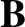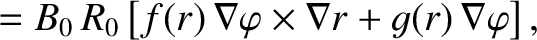(14.17)

where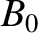is a convenient scale magnetic field-strength, and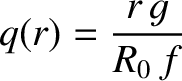(14.18)

is the safety-factor profile. [See Equation (2.128).] Here,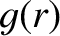and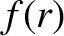are dimensionless functions.

It follows from Equations (14.1)–(14.5), (14.17), and (14.18) that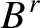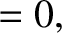(14.19)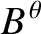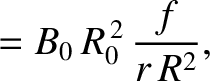(14.20)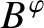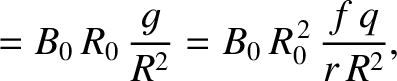(14.21)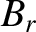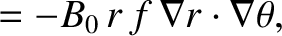(14.22)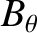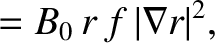(14.23)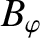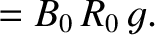(14.24)

The equilibrium poloidal magnetic flux (divided by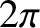),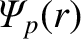, satisfies,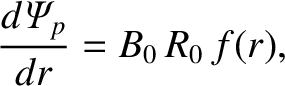(14.25)

where, by convention,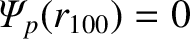. [Note that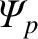is equivalent to the quantity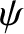defined in Equation (2.130).] The normalized poloidal magnetic flux,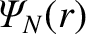, is defined such that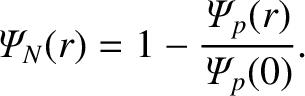(14.26)

Hence,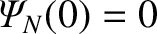and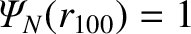. In general,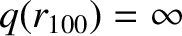. Finally, if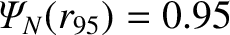then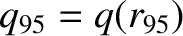. Thus,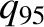is the safety-factor of the magnetic flux-surface that encloses 95% of the poloidal magnetic flux enclosed by the last closed magnetic flux-surface.